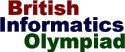The British Informatics Olympiad is the computing competition for schools and colleges. We are proud to present BIO'99.The 1999 British Informatics Olympiad - Round One

 Question 1 Digital rivers A digital river is a sequence of numbers where the number following n is n plus the sum of its digits. For example, 12345 is followed by 12360, since 1+2+3+4+5 = 15. If the first number of a digital river is k we will call it river k.For example, river 480 is the sequence beginning {480, 492, 507, 519, ...} and river 483 is the sequence beginning {483, 498, 519, ...}. Normal streams and rivers can meet, and the same is true for digital rivers. This happens when two digital rivers share some of the same values. For example: river 480 meets river 483 at 519, meets river 507 at 507, and never meets river 481. 1 (a) [20 marks] Every digital river will eventually meet river 1, river 3 or river 9. Write a program which inputs a single integer n (1<=n<=16384), and outputs the value where river n firstmeets one of these three rivers. Sample run River: 86 First meets river 1 at 101
 1 (b) [5 marks] What is the lowest number that can be found in exactly 100 digital rivers? [For example, 8 is the lowest number in 4 rivers: rivers 1, 2, 4, and 8.]

The British Informatics Olympiad